# What is Initial Transverse Metacentre?

Initial Transverse Metacentre Is defined as the point of intersection of successive lines of action of buoyancy force (Bf) when the ship is in the initial upright condition and subsequently heeled conditions.
It is assumed to be at a fixed position when the ship is heeled within small angles only.

Consider the ship shown.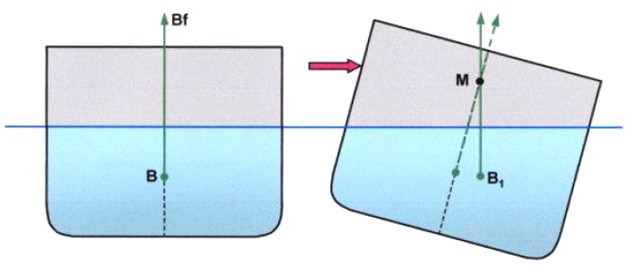When the ship heels beyond small angles the point of intersection has to move, hence the term Initial Transverse Metacentre.

It’s position is expressed as a height above the keel in metres and is termed KM. The value of KM is tabulated in ship’s hydrostatic data and its position varies with draught.

###### Calculation of KM for Box Shaped Vessel

It is convenient to consider the KM for a box-shaped vessel because the maths is simple, however, the same principles will apply for ship shapes. KM is calculated by the formula:For a box-shaped vessel on an even keel: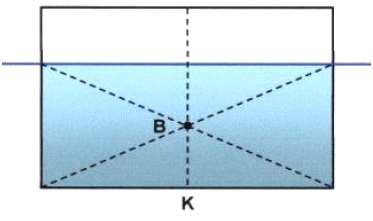BM is calculated by:where I is the moment of inertia of the water-plane area (WPA) about a longitudinal axis of rotation passing through the centre of the water-plane area; and V is the volume of displacement of the vessel.

###### Moment of inertia (second moment of area) of the water-plane area

Consider the water-plane area of a box-shaped vessel shown.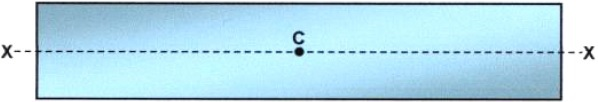C is the geometric centre of the water-plane area and XX is the longitudinal axis about which it is to be rotated.

(In reality, it is the ship that will rotate about this axis as it heels, however it is convenient to think of the water-plane area rotating about the same axis instead!)

For a box-shaped vessel: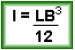where L and B are the length and breadth of the water-plane area respectively. I is in units of metres4 (m4).A simplistic, but convenient, way of considering the effect of the moment of inertia of the Water-plane area is to consider that it gives a ship resistance to heeling!
Therefore, the larger the water-plane area, the less easily a ship will heel.

If the formula for BM is considered: It is the breadth of the water-plane area that is most influential. It is generally accepted that the broader a ship is, the more stable it will be.

To summarize: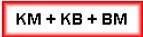and for a box-shaped vessel: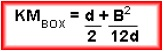error: Content is protected !!